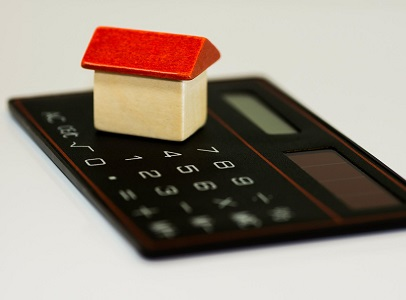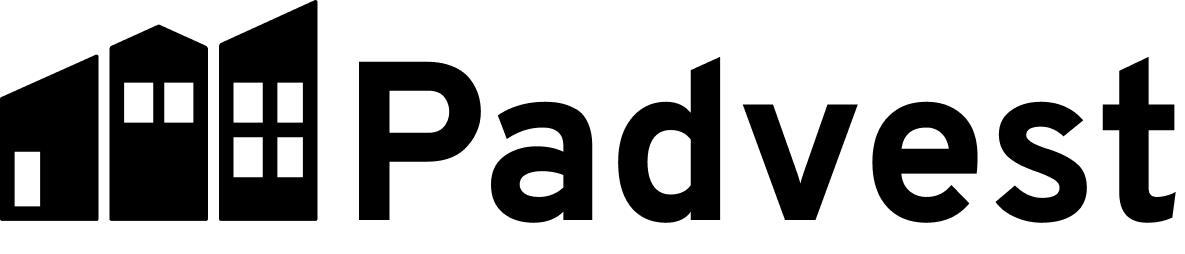# What is Cap Rate: A Real Estate Investors Guide

April 21, 2022Do you need to calculate the cap rate for a real estate investment you’re considering? Are you trying to understand how to interpret the cap rate for a particular property?

In this article we’ll answer these important questions about the Capitalization Rate (Cap Rate) so you can understand what the cap rate is, how the cap rate is calculated, how the cap rate is used to measure risk, and how to find the cap rate of a property quickly. We’ll also show you some real-world examples of how to calculate a cap rate.

## What is the Cap Rate for Real Estate?

Cap rate is a popular measure for estimating the potential profitability of a real estate investment. It is a simple metric that measures the potential rate of return for a real estate investment property. It represents the ratio of the property's expected net income to its market value. In other words, the cap rate is the percentage return you can earn on your investment, assuming an all-cash purchase.

Cap Rate should never be used as the sole indicator of an investment. It doesn’t account for your use of leverage/financing or the time value of money. It also doesn’t consider any future cash flow changes from property improvements you might make. And it doesn’t help you estimate appreciation or equity growth.

The cap rate is best used to evaluate the performance of comparable properties in the same market. It should be used in combination with longer-term metrics such as IRR (Internal Rate of Return) because on its own, the cap rate does not account for any financing, the time value of money, or future cash flow of the property. You can read about IRR and other important real estate metrics here.

Let’s look at how the cap rate is calculated, and then we’ll look deeper at using cap rate to value an investment property.

## How to Calculate the Cap Rate

For the purposes of calculating the cap rate, here are a couple of definitions:

Gross income includes rental income minus whatever vacancy allowance you may set aside as part of your strategy.

Operating expenses include all the property expenses including property taxes, insurance, HOA dues, property management, etc. And, because the cap rate assumes an all-cash purchase, the expenses for this calculation will not include debt service. Any financing will impact cash flow and the cash-on-cash return, but it is not used as part of the cap rate calculation.

Net operating income (NOI) is calculated by subtracting the operating expenses from the gross income.

Property value is similar to the present-day market value of a property. You can often start with the listing price for a property that is on the market. If you look at a cap rate using a sale price from the past, you may end up with a value that is misleading about the current cap rate of a property.

After you know the NOI and the property value, the cap rate is a simple calculation:

Cap Rate = Net Operating Income (NOI) / Property Value

By looking at the formula you can see that the cap rate is a ratio between NOI and property value. This means a higher Income can produce a higher cap rate, and a higher value can result in a lower cap rate. This will be clearer by looking at some examples of how to calculate the cap rate.

## Examples of the Cap Rate Formula

Assume that a property investor has \$500,000 in cash to invest. They find a property listed for sale for \$500,000 and it is expected to generate \$26,400 in net operating income annually. The cap rate would be 5.3%.

\$26,400 NOI / \$500,000 market value = 5.3%

If you know the estimated property value and estimated income for several properties in a neighborhood, you can determine a reasonable expected range for cap rate in that neighborhood. If you know the average cap rate you expect from a particular neighborhood, you can flip the formula to estimate an offer price for a property you are considering. With this method you can help to ensure you are not overpaying for a property.

For example, if you have determined that the average cap rate in a neighborhood is 6.3%, and the NOI is still expected to be \$26,400 you can use the formula to formulate an estimated market value – which you could use as an offer price:

\$26,400 NOI / 6.3% cap rate = \$419,050 market value

## How to Use the Cap Rate to Evaluate Investment Property

You have seen how to calculate the cap rate and how to calculate a suggested offer price for a property that is on the market if you know the area’s average cap rate. But how do you use the cap rate to evaluate a specific real estate investment to see if it is worth considering for your portfolio?

The cap rate is best used as an indicator of risk. A higher cap rate is an indicator of more risk for an investment. But a lower cap rate than the average is not necessarily a promising investment either. Let’s consider a few examples:

A property you are considering: \$26,400 NOI / \$500,000 = 5.3%
A comparable property down the street: \$34,000 NOI / \$525,000 = 6.5%
Another property in the neighborhood: \$34,000 NOI / \$425,000 = 8.0%

The average cap rate for these three properties is 6.6%. A higher cap rate is seen for a property that generates higher NOI with a lower price. A lower cap rate is seen for a property that has similar value but generates less NOI. But how can we use the cap rate to understand which property is a better investment?

Is the property with the highest cap rate the best investment? Not necessarily. It is \$100k less than the middle property and has the same income. It might be a great deal. But it also could need a new roof or some other major repair, and that could be factored into the low market value. In this example, you would want to do more research to understand why the market value is so much less than the other properties.

Is the property with the lowest cap rate better than the others? Not necessarily. It has less income and a comparable price. You would want to understand why the income was less. Perhaps it has smaller square footage, or fewer amenities. Or perhaps the value is suppressed because it is farther from public transportation, or maybe there is an opportunity to increase rent.

Remember that there is no ideal cap rate – you are evaluating cap rate so you can notice properties that are out of the average range to help you identify potential risks.

## How to Calculate and Compare Cap Rate Quickly

Padvest will help you quickly calculate the cap rate for each property – it is the first metric on the property evaluation report. Simply enter an address, customize your parameters, and see the cap rate in seconds. If you are considering multiple properties in the same neighborhood, you can evaluate each property individually, and then on the Reports page, you can see comparable property cap rates in a table view for easy comparison. You can even see the average cap rate for properties you’ve recently evaluated.